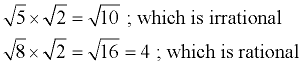Sum/Product - Rationals or Irrationals MathBitsNotebook.com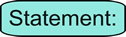"The sum of two rational numbers is rational." By definition, a rational number can be expressed as a fraction with integer values in the numerator and denominator (denominator not zero). So, adding two rationals is the same as adding two such fractions, which will result in another fraction of this same form since integers are closed under addition and multiplication. Thus, adding two rational numbers produces another rational number. Proof: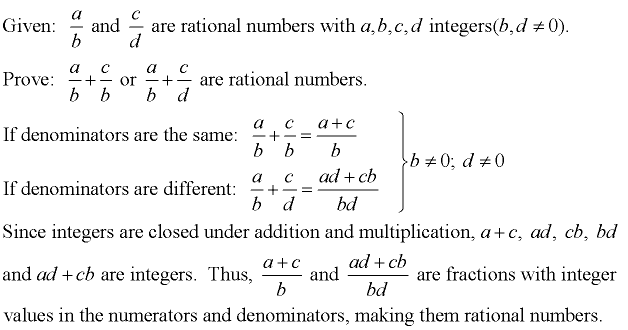"The product of two rational numbers is rational." Again, by definition, a rational number can be expressed as a fraction with integer values in the numerator and denominator (denominator not zero). So, multiplying two rationals is the same as multiplying two such fractions, which will result in another fraction of this same form since integers are closed under multiplication. Thus, multiplying two rational numbers produces another rational number. Proof: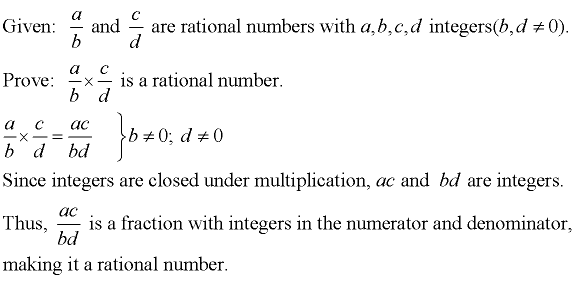Look out! This next part gets tricky!!"The sum of two irrational numbers is SOMETIMES irrational." The sum of two irrational numbers, in some cases, will be irrational. However, if the irrational parts of the numbers have a zero sum (cancel each other out), the sum will be rational.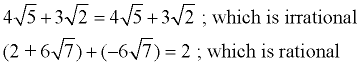"The product of two irrational numbers is SOMETIMES irrational." The product of two irrational numbers, in some cases, will be irrational. However, it is possible that some irrational numbers may multiply to form a rational product.